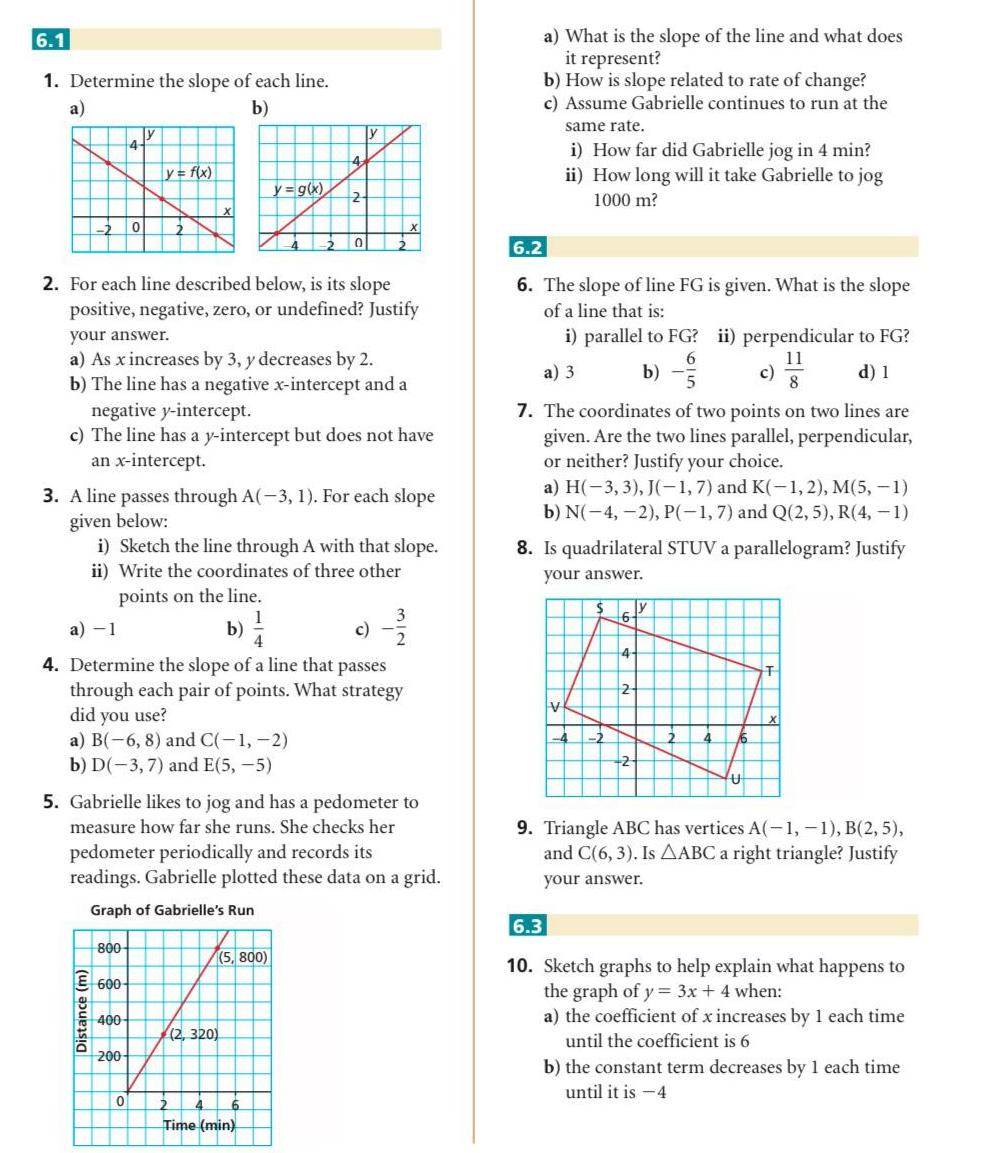Question:

# 6 1 1 Determine the slope of each line a b 4 800 E 600

Last updated: 11/20/20236 1 1 Determine the slope of each line a b 4 800 E 600 Distance m 0 y 400 y f x 200 0 X 2 For each line described below is its slope positive negative zero or undefined Justify your answer a As x increases by 3 y decreases by 2 b The line has a negative x intercept and a negative y intercept c The line has a y intercept but does not have an intercept 3 A line passes through A 3 1 For each slope given below y g x i Sketch the line through A with that slope ii Write the coordinates of three other points on the line b 14 a 1 4 Determine the slope of a line that passes through each pair of points What strategy did you use a B 6 8 and C 1 2 b D 3 7 and E 5 5 4 5 Gabrielle likes to jog and has a pedometer to measure how far she runs She checks her pedometer periodically and records its readings Gabrielle plotted these data on a grid Graph of Gabrielle s Run 2 5 800 2 320 0 y 6 Time min a What is the slope of the line and what does it represent b How is slope related to rate of change c Assume Gabrielle continues to run at the same rate i How far did Gabrielle jog in 4 min ii How long will it take Gabrielle to jog 1000 m 6 2 6 The slope of line FG is given What is the slope of a line that is i parallel to FG ii perpendicular to FG d 1 6 11 a 3 b c 8 7 The coordinates of two points on two lines are given Are the two lines parallel perpendicular or neither Justify your choice a H 3 3 J 1 7 and K 1 2 M 5 1 b N 4 2 P 1 7 and Q 2 5 R 4 1 8 Is quadrilateral STUV a parallelogram Justify your answer V 4 2 6 4 2 y T 9 Triangle ABC has vertices A 1 1 B 2 5 and C 6 3 Is AABC a right triangle Justify your answer 6 3 10 Sketch graphs to help explain what happens to the graph of y 3x 4 when a the coefficient of x increases by 1 each time until the coefficient is 6 b the constant term decreases by 1 each time until it is 4